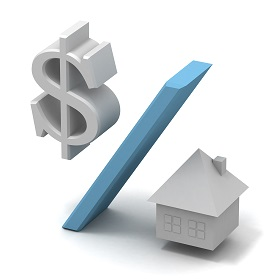## APR Calculator - Calcunation.com

Calculate the Annual Percentage Rate and how it compares with APY with this APR Calculator.

Periods per Year:
(Annual=1, Monthly=12, Weekly=52, Daily=365)
Interest Rate Per Period: